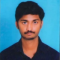## Divide:(i) $x+2x^2+3x^4-x^5$ by $2x$(ii) $y^4-3y^3+\frac{1}{2y^2}$ by $3y$(iii) $-4a^3+4a^2+a$ by $2a$Updated on 13-Apr-2023 22:54:39

## Divide:(i) $-21abc^2$ by $7abc$(ii) $72xyz^2$ by $-9xz$(iii) $-72a^4b^5c^8$ by $-9a^2b^2c^3$Updated on 13-Apr-2023 22:52:27
Given:The given expressions are:(i) $-21abc^2$ by $7abc$(ii) $72xyz^2$ by $-9xz$(iii) $-72a^4b^5c^8$ by $-9a^2b^2c^3$To do:We have to divide the given expressions.Solution:We have to divide the given polynomials by monomials using the formula $x^a \div x^b=a^{a-b}$Polynomials: Polynomials are expressions in which each term is a constant multiplied by a variable raised to a whole number power.Monomial:A monomial is an expression that contains a single term composed of a product of constants and variables with non-negative integer exponents. Therefore, (i) The given expression is $-21abc^2$ by $7abc$.$-21abc^2 \div 7abc=\frac{-21}{7}a^{1-1}b^{1-1}c^{2-1}$$-21abc^2 \div 7abc=-3a^{0}b^{0}c^{1}$$-21abc^2 \div 7abc=-3c$                    [Since $m^0=1$]Hence, $-21abc^2$ divided ... Read More

## Divide:(i) $6x^3y^2z^2$ by $3x^2yz$(ii) $15m^2n^3$ by $5m^2n^2$(iii) $24a^3b^3$ by $-8ab$Updated on 13-Apr-2023 22:51:38